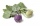# Friends

Some friends had to collect the sum 72 EUR equally. If the three refused their part, others would have to give each 4 euros more. How many are friends?

Correct result:

n =  9

#### Solution:

 n=1 e=72 (n-3).(e+4)=-152 n=2 e=36 (n-3).(e+4)=-40 n=3 e=24 (n-3).(e+4)=0 n=4 e=18 (n-3).(e+4)=22 n=5 e=14.4 (n-3).(e+4)=36.8 n=6 e=12 (n-3).(e+4)=48 n=7 e=10.2857142857 (n-3).(e+4)=57.1428571429 n=8 e=9 (n-3).(e+4)=65 n=9 **** e=8 (n-3).(e+4)=72 n=10 e=7.2 (n-3).(e+4)=78.4 n=11 e=6.54545454545 (n-3).(e+4)=84.3636363636 n=12 e=6 (n-3).(e+4)=90 n=13 e=5.53846153846 (n-3).(e+4)=95.3846153846 n=14 e=5.14285714286 (n-3).(e+4)=100.571428571 n=15 e=4.8 (n-3).(e+4)=105.6 n=16 e=4.5 (n-3).(e+4)=110.5 n=17 e=4.23529411765 (n-3).(e+4)=115.294117647 n=18 e=4 (n-3).(e+4)=120 n=19 e=3.78947368421 (n-3).(e+4)=124.631578947 n=20 e=3.6 (n-3).(e+4)=129.2 n=21 e=3.42857142857 (n-3).(e+4)=133.714285714 n=22 e=3.27272727273 (n-3).(e+4)=138.181818182 n=23 e=3.13043478261 (n-3).(e+4)=142.608695652 n=24 e=3 (n-3).(e+4)=147 n=25 e=2.88 (n-3).(e+4)=151.36 n=26 e=2.76923076923 (n-3).(e+4)=155.692307692 n=27 e=2.66666666667 (n-3).(e+4)=160 n=28 e=2.57142857143 (n-3).(e+4)=164.285714286 n=29 e=2.48275862069 (n-3).(e+4)=168.551724138 n=30 e=2.4 (n-3).(e+4)=172.8 n=31 e=2.32258064516 (n-3).(e+4)=177.032258065 n=32 e=2.25 (n-3).(e+4)=181.25 n=33 e=2.18181818182 (n-3).(e+4)=185.454545455 n=34 e=2.11764705882 (n-3).(e+4)=189.647058824 n=35 e=2.05714285714 (n-3).(e+4)=193.828571429 n=36 e=2 (n-3).(e+4)=198 n=37 e=1.94594594595 (n-3).(e+4)=202.162162162 n=38 e=1.89473684211 (n-3).(e+4)=206.315789474 n=39 e=1.84615384615 (n-3).(e+4)=210.461538462 n=40 e=1.8 (n-3).(e+4)=214.6 n=41 e=1.75609756098 (n-3).(e+4)=218.731707317 n=42 e=1.71428571429 (n-3).(e+4)=222.857142857 n=43 e=1.67441860465 (n-3).(e+4)=226.976744186 n=44 e=1.63636363636 (n-3).(e+4)=231.090909091 n=45 e=1.6 (n-3).(e+4)=235.2 n=46 e=1.5652173913 (n-3).(e+4)=239.304347826 n=47 e=1.53191489362 (n-3).(e+4)=243.404255319 n=48 e=1.5 (n-3).(e+4)=247.5 n=49 e=1.4693877551 (n-3).(e+4)=251.591836735 n=50 e=1.44 (n-3).(e+4)=255.68 n=51 e=1.41176470588 (n-3).(e+4)=259.764705882 n=52 e=1.38461538462 (n-3).(e+4)=263.846153846 n=53 e=1.35849056604 (n-3).(e+4)=267.924528302 n=54 e=1.33333333333 (n-3).(e+4)=272 n=55 e=1.30909090909 (n-3).(e+4)=276.072727273 n=56 e=1.28571428571 (n-3).(e+4)=280.142857143 n=57 e=1.26315789474 (n-3).(e+4)=284.210526316 n=58 e=1.24137931034 (n-3).(e+4)=288.275862069 n=59 e=1.22033898305 (n-3).(e+4)=292.338983051 n=60 e=1.2 (n-3).(e+4)=296.4 n=61 e=1.18032786885 (n-3).(e+4)=300.459016393 n=62 e=1.16129032258 (n-3).(e+4)=304.516129032 n=63 e=1.14285714286 (n-3).(e+4)=308.571428571 n=64 e=1.125 (n-3).(e+4)=312.625 n=65 e=1.10769230769 (n-3).(e+4)=316.676923077 n=66 e=1.09090909091 (n-3).(e+4)=320.727272727 n=67 e=1.07462686567 (n-3).(e+4)=324.776119403 n=68 e=1.05882352941 (n-3).(e+4)=328.823529412 n=69 e=1.04347826087 (n-3).(e+4)=332.869565217 n=70 e=1.02857142857 (n-3).(e+4)=336.914285714 n=71 e=1.01408450704 (n-3).(e+4)=340.957746479We would be pleased if you find an error in the word problem, spelling mistakes, or inaccuracies and send it to us. Thank you!Tips to related online calculators
Looking for help with calculating roots of a quadratic equation?
Do you have a linear equation or system of equations and looking for its solution? Or do you have quadratic equation?
Do you solve Diofant problems and looking for a calculator of Diofant integer equations?

## Next similar math problems:

• KohlrabiesThe price of one kohlrabi increased by € 0.40. The number of kohlrabies that a customer can buy for € 4 has thus decreased by 5. Find out the new price of one kohlrabi in euros.
• Area and perimeter of rectangleThe content area of the rectangle is 3000 cm2, one dimension is 10 cm larger than the other. Determine the perimeter of the rectangle.
• Isosceles triangleIn an isosceles triangle ABC with base AB; A [3,4]; B [1,6] and the vertex C lies on the line 5x - 6y - 16 = 0. Calculate the coordinates of vertex C.
• Dimensions of the trapezoidOne of the bases of the trapezoid is one-fifth larger than its height, the second base is 1 cm larger than its height. Find the dimensions of the trapezoid if its area is 115 cm2
• Lookout towerHow high is the lookout tower? If each step was 3 cm lower, there would be 60 more of them on the lookout tower. If it was 3 cm higher again, it would be 40 less than it is now.
• An equilateralAn equilateral triangle is inscribed in a square of side 1 unit long so that it has one common vertex with the square. What is the area of the inscribed triangle?
• Block or cuboidThe wall diagonals of the block have sizes of √29cm, √34cm, √13cm. Calculate the surface and volume of the block.
• The touristThe tourist wanted to walk the route 16 km at a specific time. He, therefore, came out at the necessary constant speed. After a 4 km walk, however, he fell unplanned into the lake, where he almost drowned. It took him 20 minutes to get to the shore and re
• TV competitionIn the competition, 10 contestants answer five questions, one question per round. Anyone who answers correctly will receive as many points as the number of competitors answered incorrectly in that round. One of the contestants after the contest said: We g
• Conical bottleWhen a conical bottle rests on its flat base, the water in the bottle is 8 cm from it vertex. When the same conical bottle is turned upside down, the water level is 2 cm from its base. What is the height of the bottle?
• Rectangle fieldThe field has a shape of a rectangle having a length of 119 m and a width of 19 m. , How many meters have to shorten its length and increase its width to maintain its area and circumference increased by 24 m?
• Three parallelsThe vertices of an equilateral triangle lie on 3 different parallel lines. The middle line is 5 m and 3 m distant from the end lines. Calculate the height of this triangle.
• FlowerbedWe enlarge the circular flower bed, so its radius increased by 3 m. The substrate consumption per enlarged flower bed was (at the same layer height as before magnification) nine times greater than before. Determine the original flowerbed radius.
• Sides of right angled triangleOne leg is 1 m shorter than the hypotenuse, and the second leg is 2 m shorter than the hypotenuse. Find the lengths of all sides of the right-angled triangle.
• Faces diagonalsIf a cuboid's diagonals are x, y, and z (wall diagonals or three faces), then find the cuboid volume. Solve for x=1.3, y=1, z=1.2
• Two chordsCalculate the length of chord AB and perpendicular chord BC to circle if AB is 4 cm from the center of the circle and BC 8 cm from the center of the circle.
• GP - three membersThe second and third of a geometric progression are 24 and 12(c+1) respectively, given that the sum of the first three terms of progression is 76 determine value of c